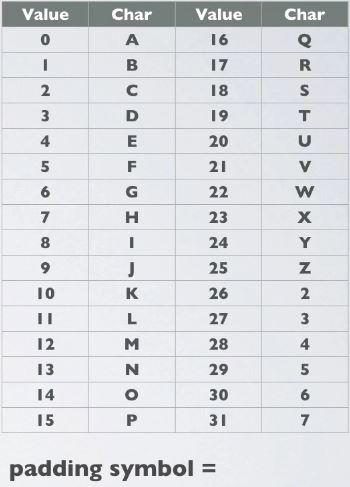# New Technology

Trending Technology Machine Learning, Artificial Intelligent, Block Chain, IoT, DevOps, Data Science## Search This Blog

• Base-32 is basically a way of encoding arbitrary binary data in ASCII text. A base-32 encoded value only uppercase letters, digits and the equal sign as padding.
• Such a value (excluding padding) can be included in an URL without encoding any characters.
• Base-32 encoding schemes uses the 26 uppercase letters A-Z, and the digits 2-7. This encoding scheme is defined in RFC 4648. The 0 and 1 are skipped due to their similarity with the letters 0 and 1.

Base - 32 Encoding

For example lets base-32 encode the word "Cat".
Cat is ASCII decimal values = [67, 97, 116]
Cat in binary format = [01000011, 01100001, 01110100]

Step 1 : Convert an input byte stream into a group of 5 bytes.
If there are less than 5 bytes, at the end, pad additional empty bytes.
Group = [01000011, 01100001, 01110100, xxxxxxxxx, xxxxxxxxxx]

Step 2. Divide this group into 8 chunks of 5 bits.
Chunks = [01000, 01101, 10000, 10111, 0100x, xxxxx, xxxxx, xxxxx]

Step 3. If a chunk has both actual end empty bits, replace the empty buts with 0's.
Chunks = [01000, 01101, 10000, 10111, 01000, xxxxx, xxxxx, xxxxx]

Step 4. Convert each 5 bits chunk to its decimal value(0-31).
If a 5 bits chunk contains empty bits replace with character '='.
Chunks = [8, 13, 16, 23, 8, =, =, =]

Step5. In the base-32 symbol chart, map each decimal value to its corresponding character.
Chunks = [I. N, Q, X, I, =, =, =]

Step 6. The word "Cat" base-32 encoded is "INQXI==="

Base - 32 Symbol Chart
• On the right is the base - 32 symbol chart.
• Lookup the decimal value in the chart and find its corresponding character in the map.
• Decimal values: 8, 13, 17, 23, 8 corresponds to: INQXI